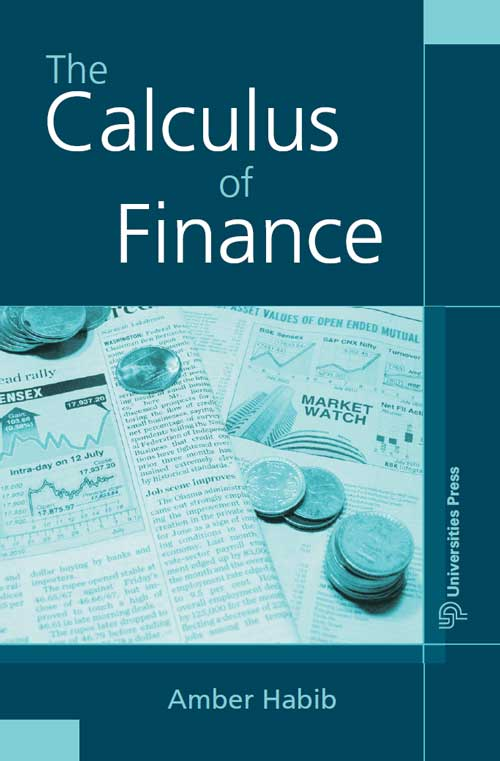## Calculus of Finance, The

#### Price: 895.00

This book is broadly about the mathematical aspects of finance. It introduces the reader to the basic concepts and products of modern finance and explores various mathematical models dealing with quantification of risk, which form the backbone of modern financial analysis. The emphasis is not so much on the details of the financial world as the basic principles by which one seeks an understanding of it. No prior knowledge of economics or finance is called for—an exposure to basic calculus and probability is all that is required of the reader. The appendix covers this ground in fair detail and would itself serve as a comprehensive primer of mathematics for finance for a beginner. The book is peppered with examples that use real-life data to ground the theory covered in the book. The exercises to be worked out are also interspersed in the text—their purpose varies from simple practice in applying formulas to extending the ideas learnt to new situations. Solutions to all the exercise problems are included as Appendix C, a feature that will be welcomed by both students and faculty. The book will serve well as an introductory book on applied mathematics in finance, of interest to students of mathematics, finance and financial management. For those starting out as practitioners of mathematical finance, this is an ideal introduction.

#### Contributors (Author(s), Editor(s), Translator(s), Illustrator(s) etc.)

Amber Habib is a professor at the Mathematical Sciences Foundation, New Delhi. He obtained his Masters in mathematics from IIT Kanpur and his PhD from the University of California, Berkeley. His research interests are in representation theory and harmonic analysis. He is also deeply involved in making mathematics education more interesting and fulfilling through special topics, projects, and an appreciation of the myriad links of mathematics with other disciplines.

1 Basic Concepts 1.1 Arbitrage 1.2 Return and Interest 1.3 The Time Value of Money 1.4 Bonds, Shares and Indices 1.5 Models and Assumptions 2 Deterministic Cash Flows 2.1 Net Present Value 2.2 Internal Rate of Return 2.3 A Comparison of IRR and NPV 2.4 Bonds: Price and Yield 2.5 Clean and Dirty Price 2.6 Price –Yield Curves 2.7 Duration 2.8 Term Structure of Interest Rates 2.9 Immunisation 2.10 Convexity 2.11 Callable Bonds 3 Random Cash Flows 3.1 Random Returns 3.2 Portfolio Diagrams and Efficiency 3.3 Feasible Set 3.4 Markowitz Model 3.5 Capital Asset PricingModel 3.6 Diversification 3.7 CAPM as a Pricing Formula 3.8 Numerical Techniques 4 Forwards and Futures 4.1 Forwards and Futures 4.2 Forward and Futures Price 4.3 Value of a Futures Contract 4.4 Method of Replicating Portfolios 4.5 Hedging with Futures 4.6 Currency Futures 4.7 Stock Index Futures 5 Stock Price Models 5.1 LognormalModel 5.2 Geometric BrownianMotion 5.3 Suitability of GBM for Stock Prices 5.4 Binomial Tree Model 6 Options 6.1 Call Options 6.2 Put Options 6.3 Put–Call Parity 6.4 Binomial Options PricingModel 6.5 Pricing American Options 6.6 Factors Influencing Option Premiums 6.7 Options on Assets with Dividends 6.8 Dynamic Hedging 6.9 Risk-Neutral Valuation 7 The Black–Scholes Model 7.1 Risk-Neutral Valuation 7.2 The Black–Scholes Formula 7.3 Options on Futures 7.4 Options on Assets with Dividends 7.5 Black–Scholes and BOPM 7.6 Implied Volatility 7.7 Dynamic Hedging 7.8 The Greeks 7.9 The Black–Scholes PDE 7.10 Speculating with Options 8 Value at Risk 8.1 Definition of VaR 8.2 Linear Model 8.3 QuadraticModel 8.4 Monte Carlo Simulation 8.5 The Martingale Appendix A: Calculus A.1 One Variable Calculus A.2 Partial Derivatives A.3 LagrangeMultipliers Method A.4 Differentiating under the Integral Sign A.5 Double Integrals Appendix B: Probability and Statistics B.1 Basic Probability B.2 Random Variables B.3 Cumulative Distribution Function B.4 Binomial Random Variable B.5 Normal Random Variable B.6 Expectation and Variance B.7 Lognormal Random Variable B.8 Cauchy Random Variable B.9 Bivariate Distributions B.10 Conditional Probability B.11 Independence B.12 Multivariate Distributions B.13 Covariance Matrix B.14 Linear Regression and Least Squares B.15 Random Sampling B.16 Sample Mean, Variance and Covariance B.17 Central Limit Theorem B.18 Stable Distributions B.19 Data Fitting B.20 Monte Carlo Simulation Appendix C: Solutions to Selected Exercises Bibliography Index

`